## 4.14Direction mixed condition

In some cases, a boundary condition is sometimes needed which applies a diﬀerent underlying type — ﬁxed value or gradient — to diﬀerent components of a non-scalar ﬁeld.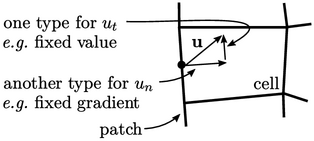The condition is most readily applied to velocity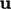, where diﬀerent conditions are applied to its normal and tangential components to the patch,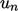and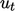respectively.

The direction mixed condition combines the mixed condition from Sec. 4.9 with the transform conditions of Sec. 4.11 . The mixed condition can be ﬁrst expressed in the form of a general transform condition Eq. (4.13 ) as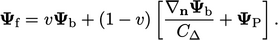(4.22)
In the direction mixed condition the value fraction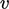is replaced by a transformation tensor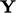, whose components are value fractions in the range 0 to 1, by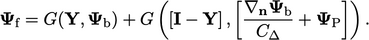(4.23)
The value fraction tensoris set according to the requirements of the boundary condition which is derived from this direction mixed framework.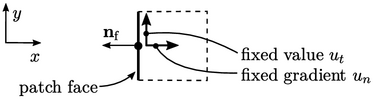Imagine an example of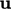at a face oriented with normal vector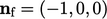, for which the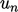condition is ﬁxed gradient and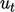is ﬁxed value. The value fraction must be 1 in the tangential direction and 0 in the normal direction, which gives: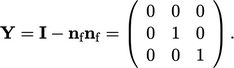(4.24)
The boundary condition is then implemented similarly to the symmetry and wedge conditions of Sec. 4.12 and Sec. 4.13 . First,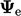is the calculated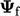using current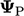by Eq. (4.23 ) and the explicit gradient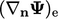is calculated from Eq. (4.17 ).

The factor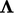corresponds to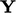. For a vector ﬁeld, it is calculated, as in the symmetry condition in Eq. (4.19 ), by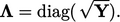(4.25)
For a tensor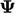, the factor is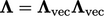, where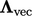are the coeﬀcients for a vector, as described for the symmetry condition in Sec. 4.12 .

The condition is implemented using value and gradient factors according to the transform condition, summarised in the table on page 284 . Any boundary condition which is based on this direction mixed condition then only requires a description of the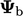,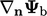and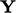parameters.

Notes on CFD: General Principles - 4.14 Direction mixed condition